Use Multiplication Gymnastics to Enhance Your Math Curriculum...An Interactive Math Activity to Help Build Multiplication Skills and Problem Solving Strategies...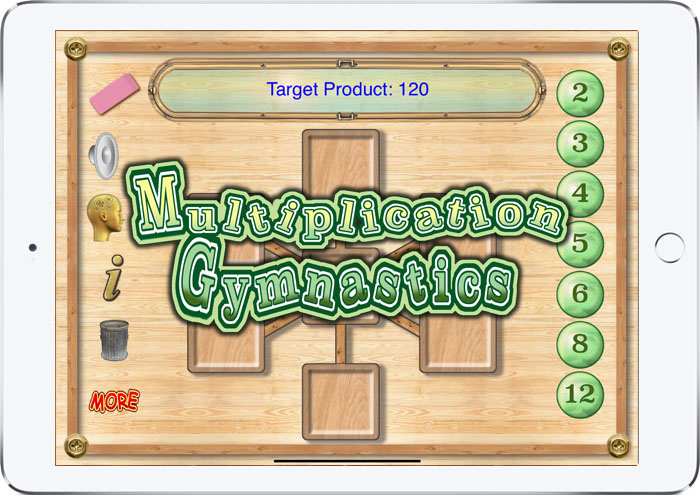Help Kids Learn Practice Multiplication Facts and Improve Basic Math Skills! The Multiplication Gymnastics app uses a puzzle format to help students see the practice multiplication facts and build problem solving strategies. This app challenges the player to find an arrangement of factors on a puzzle background where the product of the three numbers in a line equals the target product. Complete the activities by finding arranging the factors in the correct positions. The Multiplication Gymnastics app is designed to be a quick math exercise that teachers can use to provide practice with basic multiplication facts along with problem solving skills. Special sound effects make the game fun to use and the interactive design facilitates engaging learning strategies. [ See also Geometry Portal AR, Algebra Portal AR and Geometry AR ]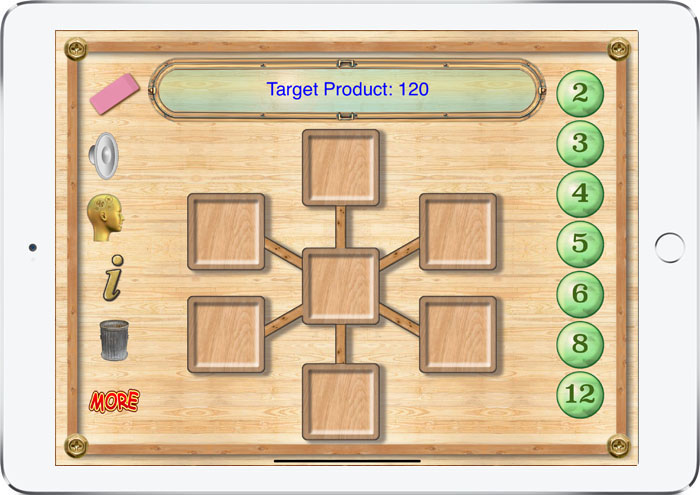The gameboard shown presents a puzzle where the object is to arrange the factors of a selected number so that when the numbers on each line are multiplied the product is equal to the given number.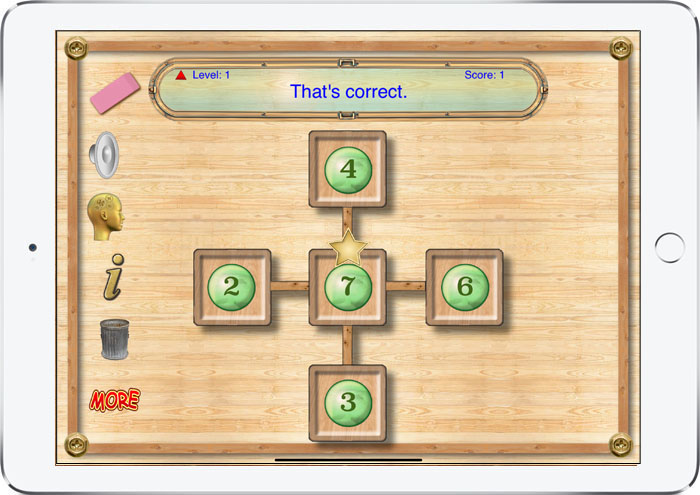Special features make using the Multiplication Gymnastics app fun: 1. Slide to place the factors in an arrangement that makes the given product. 2. Random problems are automatically generated. 3. Speech and sound effects. 4. Available for iPad iOS.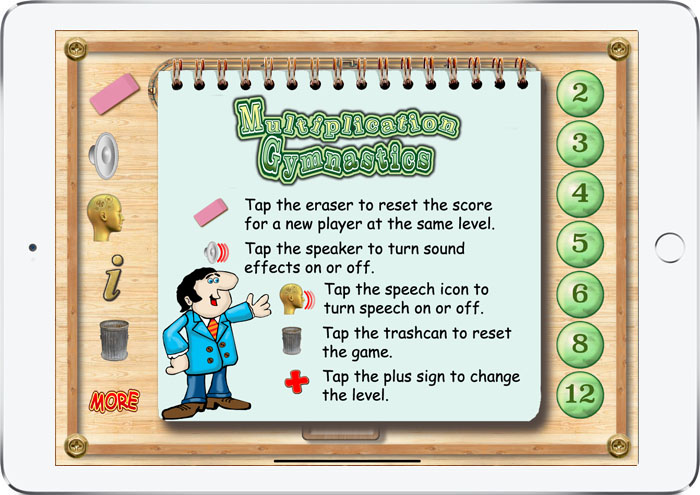Available now for Apple TV Bring the excitement of exploring the multiplication facts and factorization using Multiplication Gymnastics in your classroom or living room with the Apple TV version of the Multiplication Gymnastics.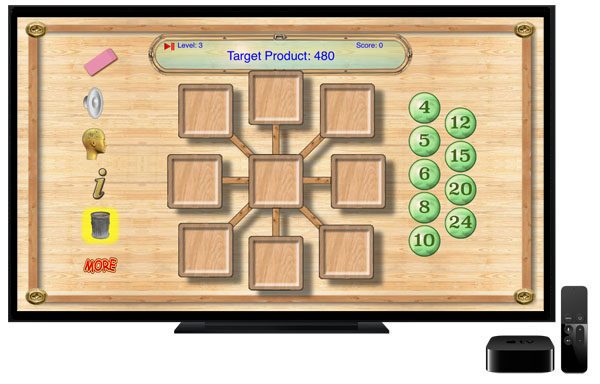FREE! Now Available on the Apple App Store.